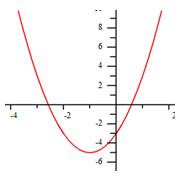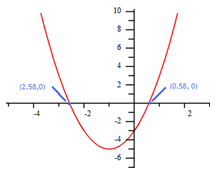Solutions
Example

Find the solutions to the equation 2x2 + 4x - 3 = 0 by graphing.

Step 1. Rewrite the equation by substituting a y in the place of the zero.

y = 2x2 + 4x - 3

Step 2. Use a graphing calculator to make a graph of the function.Step 3. Does the graph have two, one or no x-intercepts (solutions)?

This graph has 2 x-intercepts, therefore look for 2 solutions to the equation.

Step 4. Use the calculate zeros command to calculate the solutions (x-intercepts) of the equation.*Note: Sometimes the solutions will appear on the table. Use the table function of a graphing calculator and find the values on the table where the y -coordinate is zero.